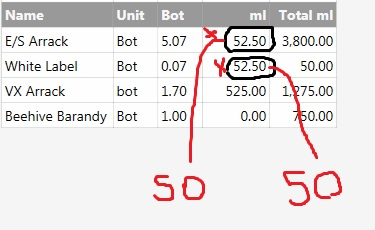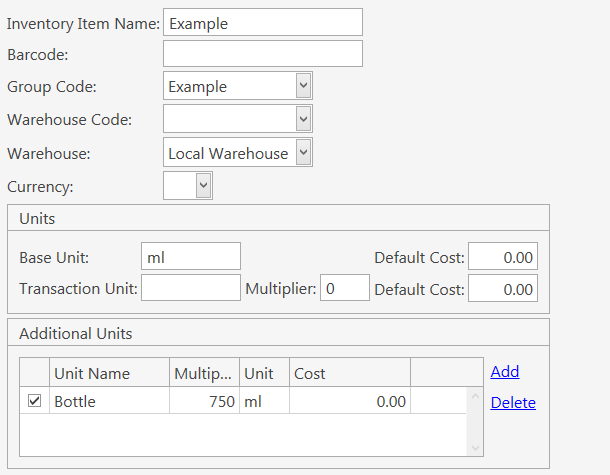# Anyone Can Hell Me Inventory consumption Report Calculate "C.Consumption.decimal * 750"

Blockquote
[Consumption:5,2,2, 3, 3]

Name|Unit|Bot|ml|Total ml

{REPORT CONSUMPTION DETAILS:C.Name,C.Unit,[C.Consumption.Sum],[([C.Consumption])-([C.Consumption]).Sum],[C.Consumption]*750:C.Consumption != 0}\

ml = C.Consumption.decimal * 750
ex :- 0.7 * 750 = 525

Can you explain why you want to calculate the decimal amount?

E/S Arrack Bottle consumption is 5.57

E/S Arrack Bottle =750ml

So i need to calculate .57 for ml amount

Then i need to get .57

E/S Arrack Bottle 5
E/S Arrack ml 427.5 ml

Try this:

``````[Consumption:5,2,2, 3, 3]
Name|Unit|Bot|ml|Total ml
{REPORT CONSUMPTION DETAILS:C.Name,C.Unit,[C.Consumption.Sum],[([C.Consumption])-([C.Consumption]).Sum],[C.Consumption]*750:C.Consumption != 0:{0}|{1}|[=F(TN('{2}'),'0000.00').substr(5,2)*.750]|{3}|{4}}
``````

What’s happening:

`[=F(TN('{2}'),'0000.00')` This will convert any number to 4 whole numbers, a period then 2 decimal places. For example a number of `5` will be converted to `0005.00`.

`.substr(5,2)` This is used to select character(s). Starting at the first zero (counting from 0…not 1) we going to count until we get to the first decimal place. In this case it’s in the 5th position. Then we want to select 2 characters, starting on the first decimal number(the 5th position from the previous step) we are going to count 2 places (counting from 1…not 0 this time). We could also use `substr(6,-2)` and get the same results. This will select the 2 decimal places.

If you think you will have a consumption more than 9999, you will have to adjust the formats accordingly.

Edit

This is a solution by subtracting the value with decimal - value as integer and multiplying by 750.

``````[Consumption:5,2,2, 3, 3]
>Name|Unit|Bot|ml|Total ml
{REPORT CONSUMPTION DETAILS:C.Name,C.Unit,[C.Consumption.Sum]:C.Consumption != 0:{0}|{1}|{2}|[=F((TN('{2}')-parseInt(TN('{2}')))*750),'#.##']|[=F((TN('{2}')*750)),'#.##']}``````
1 Like
``````indent preformatted text by 4 spaces
``````

[Consumption:5,2,2, 3, 3]

Name|Unit|Bot|ml|Total ml
{REPORT CONSUMPTION DETAILS:C.Name,C.Unit,[C.Consumption.Sum],[C.Consumption]*750:C.Consumption != 0:{0}|{1}|{2}[=F(TN(’{2}’),‘0000.00’)750]|[=F((TN(’{2}’)- parseInt(TN(’{2}’)))*750),’#.###’]|{3}}

But Its Not CorrectIts Not Correct
Can You Help Me

That is not how math works. It is correct.

`.07 * 750 = 52.50 `

Actually, after thinking about this some more, you are going to want the whole number and decimal.

Lets say you have a 1.5 bottles of Arrack. Each bottle holds 750 ml. You are going to have a total of 1125 ml of Arrack (1.5 bottles * 750 ml = 1125 ml). The way you want to figure it, will show only 375 ml (.5 bottle * 750 ml = 375 ml). It will be off 750 ml (the ml’s of 1 bottle).

``````    1 bottle * 750 ml = 750 ml
+ 0.5 bottle * 750 ml = 375 ml
----------------------------------------
1125 ml
``````

Yes Bob_be

But the think is he sale 50ml,100ml,375ml,750ml
So if you think he sale 50ml shoot
50/750=0.066666667

Then system shows 0.07 Bottle

If we call calculate this way
0.066666667 * 750 = 50 ml

I need to do like this

Now you send report sho like this
0.07 * 750 =52.5 ml
This is not correct aunser

Can you help me to crate a good report375ml and 750 ml is okkk
But
50 ml and 100ml calculate is not correct

50ml =0.06666667
100ml = 0.13333333

This is the decimal places i need to calculate

ThanksSo change the decimal places in your tn to 000.00000000 or whatever you need.
Normal practice is you set your inventory unit as the lowest common denominator.

So change #.## to the precision you want, or use as a principal unit 50 ml…

Ok, I understand what you are saying now.

One thing we have to understand, is that inventory numbers will almost always not match physical inventory exactly, when dealing with pourable liquids or measurable ingredients. If a POS system says inventory of 1.25, it would be reasonable to think that the physical inventory is 1 full bottle with another bottle that is somewhere in between 1/2 and almost empty. The failing point is not the POS system but rather us people.

The issue is rounding…You can try extending the decimals as JTRTech and Francisco_Lopez mentioned. You will have to also `.substr` accordingly.

Maybe another option would be figure out how many 50 ml serving you have???

1 Like

This is what I mean about lowest common denominator as inventory unit.
Using ml as unit would make life allot easier, 50 won’t work with 375ml so either 25ml or 1ml base unit, I’d say 1ml as clean accross the board unit rather than say 25ml which fits in all sizes but less clear to work with.

As Bob says though, I’ve never seen a stock take calculate as accurate as single shot. Our stocktaker does 10th of a bottle as count unit for closing stock but even experianced stocktaker won’t get that 100% accurate meaning typical variance arround 2-4 shots on monthly stocktake is within acceptable margin of error.
Unless your weighing or decanting to measure your not going to be acurate to much under 50ml anyway with different shape bottles etc.
Ml unit keeps math and recipe simple.
If you work on bottle as you say a recipe for a shot is already 0.06666666667 which is odd way to work in my opinion.

1 Like

Every Bottle have 750ml, 375ml, 100ml and 50ml

Report is not correct so i try different way

But results is not good

System round bottle amount
System shows
Bottles 1.07

Real Bottle 1.06666667

ThanksIs that .0001 difference really gonna matter? I don’t understand.

``````[Consumption:5,2,2, 3, 3]
>Name|Unit|Bot|ml|Total ml
{REPORT CONSUMPTION DETAILS:C.Name,C.Unit,[C.Consumption.Sum]:C.Consumption != 0:{0}|{1}|{2}|[=F((TN('{2}')-parseInt(TN('{2}')))*750),'#.##########']|[=F((TN('{2}')*750)),'#.###########']}
``````

50ml and 100ml Is not Correct

I Put Vat 69 50ml Shoot

Correct Answer Is 50 But System Show It 52.50

Any One Can Help Me to Get Correct Answer

Then modify inventory items, set base unit as ml and add an aditional unit called Bottle with a multiplier of 750 ml.Then modify recipes in order to match the new setup:

Your setting decimal places after the calculation. If origional figues already rounded to 2 then your math isn’t going to just put back the rounding at the end.
BTW just keep saying its wrong isn’t very productive.

Decimal places may not even help if samba is only storing #.## in dB, likely need to adjust a setting somewhere to increase, posibly on windows regional settings.

Either way multiple times you have been told to change your base unit, id sugest doing that, lowest common denominator is common method and suggested many times on multiple threads.

[Consumption:5,2,2, 3, 3]

Name|Unit|Bot|ml|Total ml
{REPORT CONSUMPTION DETAILS:C.Name,C.Unit,C.Consumption.Sum:C.Consumption != 0:{0}|{1}|[=F(’{2}’,’#’)]|[=F((TN(’{2}’)-parseInt(TN(’{2}’)))*750,’#’)]|[=F((TN(’{2}’)*750),’#’)]}

Done

1 Like

1 st Decimal Place Change
Manage > Settings > Program Settings Currency Decimals > Visible Decimal Place = " 3 "

``````[Consumption:5,2,2, 3, 3]
>Name|Unit|Bot|ml|Total ml
{REPORT CONSUMPTION DETAILS:C.Name,C.Unit,C.Consumption.Sum:C.Consumption != 0:{0}|{1}|[=F(parseInt(TN('{2}'),'#'))]|[=F((TN('{2}')-parseInt(TN('{2}')))*750,'#')]|[=F((TN('{2}')*750),'#')]}
``````

Report

Thanks Help me
Bob_be, Francisco_Lopez, JTRTech,Jesse,Memo,

1 Like